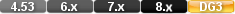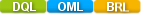Easy to Create, Easy to Change - Easy to use!## FloorFloor(NUMERIC VALUE) Floor(123.45) Floor(MyNumericalField) Floor(MyField1*VAT+TravelExpenses)

The floor function rounds down a numeric value to the next lowest integer.

Basically it strips off the decimals and leave an integer.

The numeric value in a Math function can be a constant value, a variable, a field value, or an expression

# Parameters

Numeric Value. The numeric value in a Math function can be a constant value, a variable, a field value, or an expression

Intger value.

Examples

floor( 5.000)

Returns: 5

floor( 5.999)

Returns: 5

# floor

Math Function

#### Purpose

The floor function rounds down a numeric value to the next lowest integer.

Syntax

floor( NUMERIC VALUE)

Returns

An integer value.

Usage

The numeric value in a Math function can be a constant value, a variable, a field value, or an expression.

Examples

floor( 5.000)

Returns: 5

floor( 5.999)

Returns: 5

floor ( ( current date - BIRTHDATE) /365.25)

Returns: The result of the age calculation. The operation contained in the interior set of parentheses is performed first and returns an age value expressed in days. The exterior calculation converts the age in days to years. If the current date is 05/30/2001 and the value in the BIRTHDATE field is 07/02/62, the function returns 38.